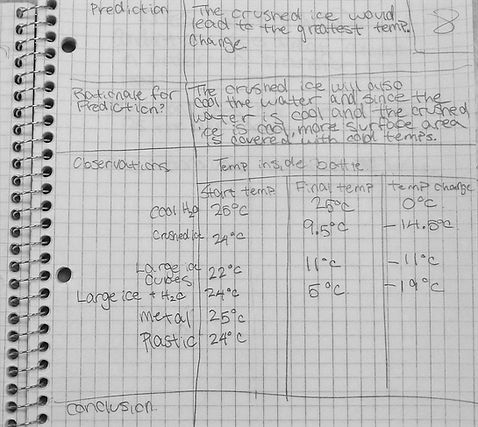Engineering Knowledge: Engineering Sciences

## Engineering Performance Matrix

Thermodynamics is the science of transferring energy from one place or form into another place or form which includes the study of heat and temperature and the relation of these factors to work, energy, and power. This concept is important to Engineering Literacy, as it is the basis on which engineering professionals calculate and predict how forms of energy are converted into other forms in order to create, improve, and create technological products and systems such as power plants, air-conditioning/heating units, and automobile engines.

## Performance Goal for High School Learners

I, when appropriate, draw upon the knowledge of Thermodynamics content, such as (a) the Laws of Thermodynamics, (b) equilibrium, (c) gas properties, (d) power cycles and efficiency, and (e) heat exchangers, to analyze the forces within an energy system to solve problems in a manner that is analytical, predictive, repeatable, and practical.

## THERMODYNAMIC PROPERTIES, LAWS, & PROCESSES

I can identify the basic properties and behaviors of heat.

## EQUILIBRIUM

I can define thermal equilibrium, describing its basic conditions.

I can explain the four laws of thermodynamics (e.g. Zeroth, First, Second, and Third laws).

I can explain the ideas case where a system is in an equilibrium state, referring to the second law of thermodynamics.

I can discuss the thermodynamic process in a certain situation.

I can analyze equilibrium states of a thermodynamic system, using mathematical equations and correct units.

## GAS PROPERTIES

I can describe the basic properties of gas (e.g. pressure, volume, number of particles, and temperature).

I can explain the variables determining each of gas properties.

I can analyze the properties of a gas, using mathematical equations and correct units.

## POWER CYCLES & EFFICIENCY

I can define thermodynamic power cycles.

I can explain different types of power cycles (e.g. ideal, Carnot, and Stirling cycles) in terms of their compositions and thermal efficiency.

I can analyze the performance of a given thermodynamic cycle, using mathematical equations and correct units.

## HEAT EXCHANGERS

I define the functions and applications of heat exchangers.

I can explain different types of heat exchangers in terms of their flow arrangement.

I can determine and justify which type of heat exchangers is most appropriate to a given design problem.